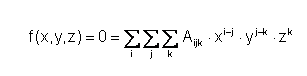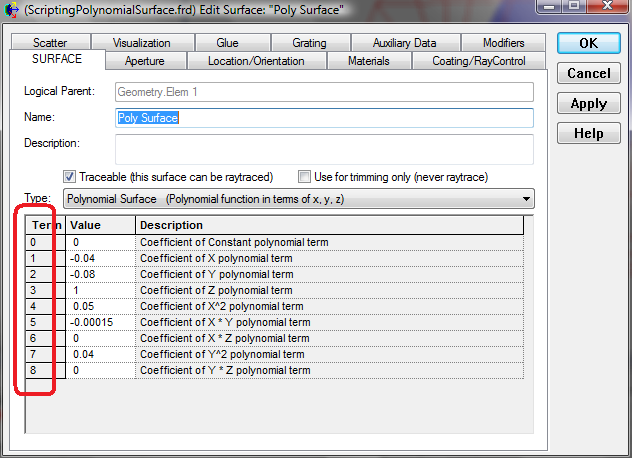# Scripting a Polynomial Surface

The Polynomial Surface Type has a general form and can therefore be used for a wide variety of surface shapes.This surface can be created using the scripting language using the AddPolynomialSurf function which requires the T_ENTITY structure (the fundamental information of the surface object such as the name, its parent etc.) and an array of coefficients. The key part of the script is to understand how to provide the coefficients of the polynomial.

Although the equation described above is a function of i,j,z, the AddPolynomialSurf function expects a one dimensional array where the coefficients are in the order as shown in the GUI for the surface. The index shown in the Term column matches the array index.The example script below will create a Polynomial Surface with the following form:

-0.04*X - 0.08*Y + 0.05*X^2 -0.00015*XY + 0.04*Y^2

```    Dim ent As T_ENTITY, id1 As Long, id2 As Long

InitEntity ent

'Custom Element parent
ent.name = "Elem 1"

'polynomial surface as child of Custom Element
ent.name = "Poly Surface"
ent.parent = id1

Dim coefs(7) As Double

coefs(0) = 0        'A
coefs(1) = -0.04    'X
coefs(2) = -0.08    'Y
coefs(3) = 1        'Z
coefs(4) = 0.05     'X^2
coefs(5) = -0.00015 'XY
coefs(6) = 0        'XZ
coefs(7) = 0.04     'Y^2

id2 = AddPolynomialSurf ( ent, coefs )

'Need to make the Boundary Box bigger...
Dim trimVol As T_TRIMVOLUME
GetTrimVolume id2, trimVol
trimVol.xSemiApe = 2
trimVol.ySemiApe = 2
trimVol.zSemiApe = 2
SetTrimVolume id2, trimVol

'Final UPDATE
Update<br>
```